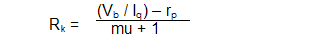Neophytes are often horrified by 5% differences between a circuit's actual functioning and the calculated results; whereas a seasoned circuit designer would revel in such exactitude.
 advantage of using the actual triode's characteristics, but requires a good eye and some skill; worse still, not all triodes find their corresponding plate curves in print.    Fourth, track down the value by actual experimentation. This requires both some physical work and some expense, as the triodes and resistors must be purchased and several triodes should be tested to preclude having a gassy or a weak triode throw off the results.     And fifth, use a SPICE program to determine the value.  This is actually something of a combination of the first four approaches. The limitation here is that the result is only as good as the SPICE models of the triode used and most of the models I have seen are just okay -- and there are not that many of them out there (far fewer in number than the number of published plate curves) -- but the models are steadily improving and growing in number.       For most tube circuit designers the methods that work best are the second (working out mathematically) or third (inspecting the plate curves) because these methods quickly give reasonably accurate results.  The formula for determining the cathode resistor's value (in the absence of a plate resistor) is a simple one:
 While vacuum tubes are much more consistent than other discrete active devices such as transistors, MOSFETs, and FETs, if you want tight tolerances, then look to passive devices, as 0.01% tolerances only exist in passive components such as resistors and capacitors.     Still, why so large a difference? Part of the answer is found in the formula's assumption of a perfect triode. Another part is found in the tube manual's imprecision in stating the mu and the rp of the 6SN7 which, in this case, forms the larger part of the error. A careful inspection of the plate curves reveals that at 250 volts and 9 mA, the mu is closer to 21 and the rp is closer to 8k or 9k. If we recalculate the cathode resistor's value using these revised values, then much of the difference disappears between formula and plate curves.    Aren't a triode's mu and rp fixed and immutable? No. Unlike a tube's dimensions and mass, its rp, mu, and Gm vary, depending on plate voltage and plate current. The least varying characteristic is its mu; which is ironic, as it is the least real of the three specifiers, defining only the relation between rp and Gm, not any actual physical aspect of the triode's design. (Naming the ratio between a man's height and the circumference of his waist "phi" might be useful for predicting heart attacks, but phi is not real in the same way that his eyes or kidneys are real.)     Often, most of the blame for inaccurate results lies with the difference in cathode-to-plate voltage that the tube manual specifies versus the voltage the formula assumes. The tube manual assumes that fixed bias will be used, thus retaining the full B+ across the tube, whereas the formula accounts for the voltage lost across the cathode resistor.where, Rk equals the cathode resistor; Vb, the B+ voltage; Iq, the desired idle current; rp, the plate resistance; and mu, the triode's amplification factor. (If the result is a negative number, then the desired idle current requires a lower rp triode.) For example, a 6SN7 (rp = 7700, mu = 20) with B+ voltage of 250 volts and  a desired idle current of 9 mA will need a cathode resistor of  956 ohms, which against the 9 mA idle current equals 8.6 volts and is close to the tube manual's 8 volts. In fact, in actual practice, this value would probably be close enough, or at least close enough to allow easy fine tuning. (Adding a plate resistor to the circuit will also serve to promote consistency in idle current and its value comes from the voltage drop divided by Iq.)